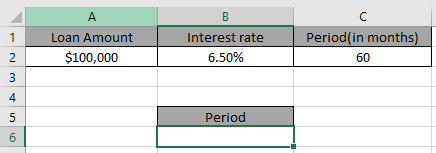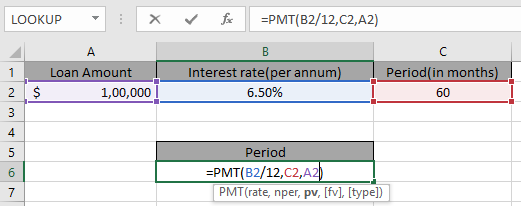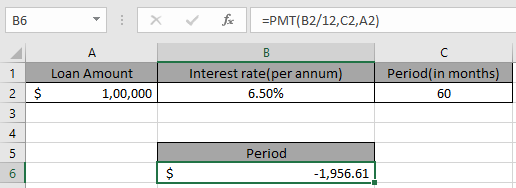# How to calculate interest on a loan in Excel

In this article, we will learn how to use the PMT function to calculate loan on payments in Excel.

Excel lets a person find monthly instalment on a loan amount using the function having principle amount or loan amount, interest rate per month and the period of payment.

The function calculates the payment for a loan based on constant payments and a constant interest rate.
Syntax:

=PMT (rate, nper, pv, [fv], [type])

Syntax for loan calculation formula:-
Rate – The interest rate per period.
Per – The period for which the interest rate is calculated.
Nper – The total number of payments.
Pv – The present value, the total amount that a series of future payments is worth now.
Type – The timing of the payment, either at the beginning or end of the period. Numbers 0 or 1 represent the payment date. The number 0 represents payment at the end of the period, and the number 1 represents payment at the beginning of the period. The default (empty argument) is 0. The calculation is at the end of the period.

All of the above might be confusing for some people, so let’s gear up & start learning its usage in excel with the example.

Consider a scenario, John has taken a loan of \$100,000 from a bank at 6.5% per year interest in 5 years period. He needs to find out his monthly payment to the bank paying his loan.

So he added the following details in Excel.Use the formula in B6 cell

=PMT(B2/12,C2,A2)You must be wondering why Interest rate is divided by 12 as Applying the formula in the cell.John has to pay \$1,956 per month. You must be wondering why this amount shown here is negative. The loan amount is positive which is credited to John. He has to pay that amount which is to be debited.

You can use minus sign(-) in the formula to get the result in positive sign(+)
Use the alternative formula for signs

=PMT(B2/12,C2, -A2)

Hope you understood how to calculate monthly installments on a loan using PMT function. Explore more articles here on calculating accounts function here. Please state your queries in the comment box below.

Popular Articles:

50 Excel Shortcuts to Increase Your Productivity

How to use the VLOOKUP Function in Excel

How to use the COUNTIF function in Excel

How to use the SUMIF Function in Excel

1.I would like to know how to create a spreadsheet for varying payments on a loan with interest.

I have looked at amoritization templates, but they don't allow for entering adjusted payments (higher or lower than the payment due). For example, overpayments.

2.I would like to know how to calculate fortnightly payment in excel.

•Hi,

Thanks for contacting us!

You can go through http://www.excelforum.com/excel-formulas-and-functions/354668-fortnight.html with this link or you can ask query @ www.excelforum.com.

Thanks
Excel Forum & Excel tip team

3.I want to how to calculate fortnightly payment is excel

4.i would like to have guide for calculate

5.i want to calculate work time and work OT time for employees in factory

Terms and Conditions of use

The applications/code on this site are distributed as is and without warranties or liability. In no event shall the owner of the copyrights, or the authors of the applications/code be liable for any loss of profit, any problems or any damage resulting from the use or evaluation of the applications/code.